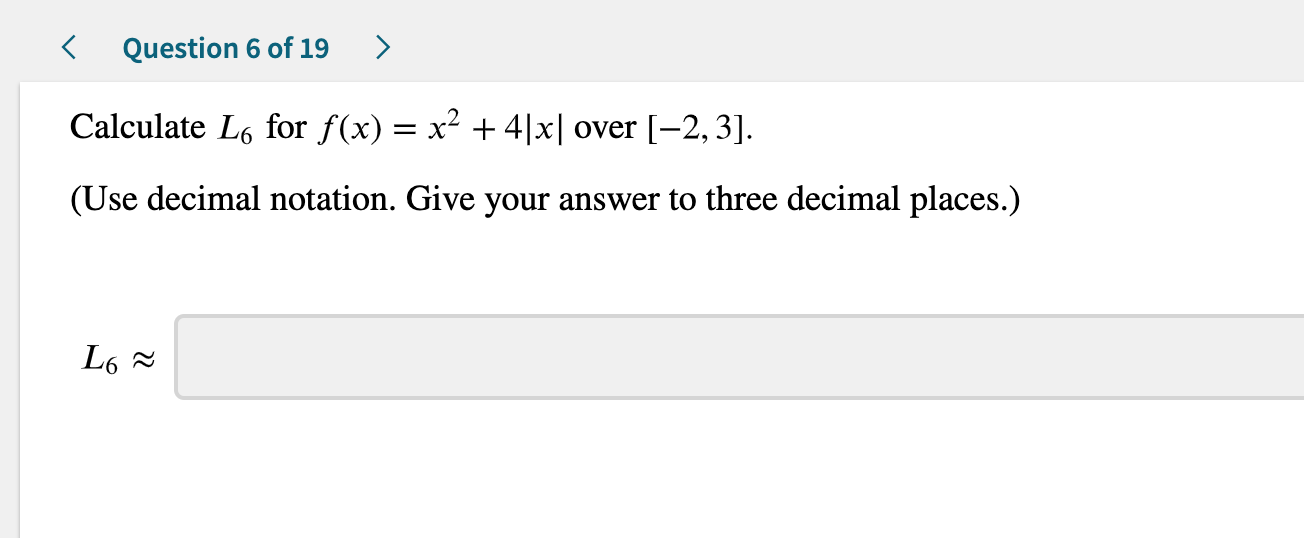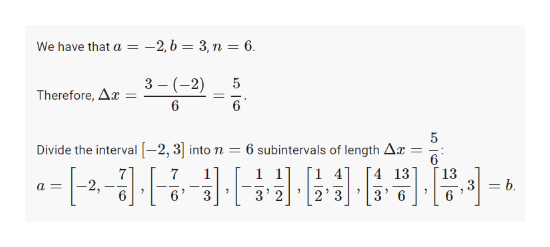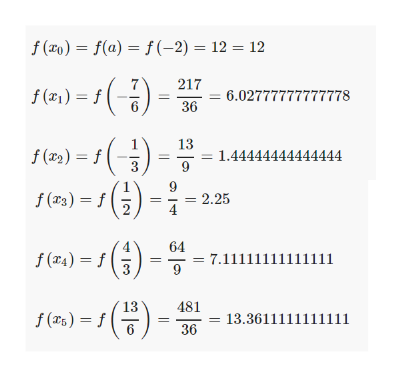# Question 6 of 19Calculate L6 for f(x) = x2 +4|x| over [-2,3](Use decimal notation. Give your answer to three decimal places.)L6

Question
5 viewshelp_outlineImage TranscriptioncloseQuestion 6 of 19 Calculate L6 for f(x) = x2 +4|x| over [-2,3] (Use decimal notation. Give your answer to three decimal places.) L6 fullscreen
check_circle

Step 1

First find the intervals.help_outlineImage Transcriptionclose-2,b = 3,n = 6 We have that a 3 (2) 6 5 Therefore, Ar= 6 Divide the interval-2, 3into n 6 subintervals of length Ar 6 [4 13 13 7 1 2, 6 = b a= 2' 3 6 fullscreen
Step 2

Now, we evaluate the function at ...help_outlineImage Transcriptionclosef (xo)= f(a) = f(-2) 12 12 217 f(2)f 6.02777777777778 36 13 1.44444444444444 f (x)=f f (r3)=f 2.25 4 64 f (r4)=f 7.11111111111i11 13 481 13.3611111111111 6 36 fullscreen

### Want to see the full answer?

See Solution

#### Want to see this answer and more?

Solutions are written by subject experts who are available 24/7. Questions are typically answered within 1 hour.*

See Solution
*Response times may vary by subject and question.
Tagged in

### Other